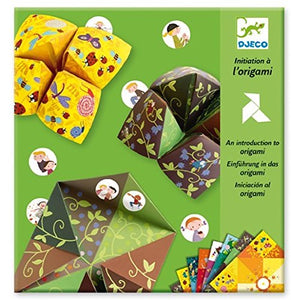# Djeco Origami - Bird Game

Papo

• \$18.53
Unit price per
• Save \$12.98

• T

• h

• i

• s

• k

• i

• t

• c

• o

• n

• t

• a

• i

• n

• s

• 2

• 4

• o

• r

• i

• g

• a

• m

• i

• p

• a

• p

• e

• r

• s

• ,

• 3

• s

• h

• e

• e

• t

• s

• o

• f

• s

• t

• i

• c

• k

• e

• r

• s

• a

• n

• d

• a

• f

• u

• l

• l

• c

• o

• l

• o

• u

• r

• i

• n

• s

• t

• r

• u

• c

• t

• i

• o

• n

• b

• o

• o

• k

• l

• e

• t

• .

Djeco have created a fun, modern version of the popular playground game of 'Chooses' The kit includes lots of beautifully patterned origami papers with simple instructions on how to fold the papers to form the familiar game. There are also lots of illustrated stickers which can be used to mark each section with various challenges for the players .... such as pretend to ride a horse or jump as high as you can! Children love this game - it will keep them entertained for hours!